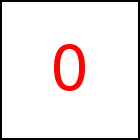# rotate Library Method

Short Description: Rotate around given point and axis

Signature: t.rotate (px,py,pz, ax,ay,az)
Group: Motion
Class: transition Class

Makes the object rotate around the point (px,py,pz) with the axis (ax,ay,az). (px,py,pz) denote the Coordinates of the center point of the rotation. px..az must be numbers which can be integer or floating point. The vector (ax,ay,az) must be a unit vector, i.e. it must have length 1.

For example (ax,ay,az)=(0,0,1) makes the object rotate around the z-axis, i.e. inside the screen plane.

Per default a full rotation of 360 dregrees is performed. This can be changed using the rotatePart function. In order to rotate an object around its center refer to rotateMiddle.

A transition may perform a single rotation and a single motion with constant acceleration. So only of the rotate or rotateMiddle methods may be called once per transition. If a motion with constant acceleration is defined as well, then the center point (px,py,pz) refers to the end point of the motion with constant acceleration. So conceptually the object is first drawn at the end point, then rotated, and then moved to the point where it belongs w.r.t. the motion with constant acceleration.

### Examples

 var a=taccgl.actor("testimg"); a.rotate(a.x0,a.y0,0,0,0,1).start(); RUN var a=taccgl.actor("testimg"); a.rotate(a.x0,a.y0+a.hy0,0,1,0,0).start(); RUN var a=taccgl.actor("pgMiddleColumnTable"); a.rotate(500,500,500,0,1,0).start(); RUN var a=taccgl.actor("pgMiddleColumnTable"); a.rotate(500,500,-500,0,1,0).start(); RUN var a=taccgl.actor("pgMiddleColumnTable"); a.rotate(500,500,-500,1/Math.sqrt(2),1/Math.sqrt(2),0).dur(5).start(); RUN var a=taccgl.actor("pgMiddleColumnTable"); a.rotate(500,500,-500,1,1,0).dur(5).start(); RUN

WebGL™ is a trademark of the Khronos Group Inc.

Next Page:transition.rotateMiddle - Rotate around Objects Middle and given axis
Previous Page: transition.vEnd - Set final velority